SSC BOARD PAPERS IMPORTANT TOPICS COVERED FOR BOARD EXAM 2024

# Work And Energy

##### Class 9th Science & Technology MHB Solution
Exercises
1. Explain the difference between potential energy and kinetic energy. Write detailed…
2. Derive the formula for the kinetic energy of an object of mass m, moving with velocity v.…
3. Prove that the kinetic energy of a freely falling object on reaching the ground is nothing…
4. Determine the amount of work done when an object is displaced at an angle of 30^0 with…
5. If an object has 0 momentum, does it have kinetic energy? Explain your answer. Write…
6. Why is the work done on an object moving with uniform circular motion zero? Write detailed…
7. For work to be performed, energy must be …. i transferred from one place to another ii…
8. Joule is the unit of … i. force ii. work iii. power iv. energy Choose one or more correct…
9. Which of the forces involved in dragging a heavy object on a smooth, horizontal surface,…
10. Power is a measure of the ...…. i. the rapidity with which work is done ii. amount of…
11. While dragging or lifting an object, negative work is done by i. the applied force ii.…
12. The potential energy of your body is least when you are ….. i. sitting on a chair ii.…
13. The total energy of an object falling freely towards the ground … i. decreases ii. remains…
14. If we increase the velocity of a car moving on a flat surface to four times its original…
15. The work done on an object does not depend on …. i. displacement ii. applied force iii.…
16. 1. Take two aluminium channels of different lengths. 2. Place the lower ends of the…
17. 1. Take two aluminium channels of different lengths. 2. Place the lower ends of the…
18. 1. Take two aluminium channels of different lengths. 2. Place the lower ends of the…
19. 1. Take two aluminium channels of different lengths. 2. Place the lower ends of the…
20. 1. Take two aluminium channels of different lengths. 2. Place the lower ends of the…
21. An electric pump has 2 kW power. How much water will the pump lift every minute to a…
22. If a 1200 W electric iron is used daily for 30 minutes, how much total electricity is…
23. If the energy of a ball falling from a height of 10 metres is reduced by 40%, how high…
24. The velocity of a car increase from 54 km/hr to 72 km/hr. How much is the work done if the…
25. Ravi applied a force of 10 N and moved a book 30 cm in the direction of the force. How…
26. Study the various instances of transformation of energy you see around you and discuss…

###### Exercises
Question 1.

Explain the difference between potential energy and kinetic energy.

Kinetic energy and Potential energy are the two forms of energy which any object or body have, they are different from each other in following ways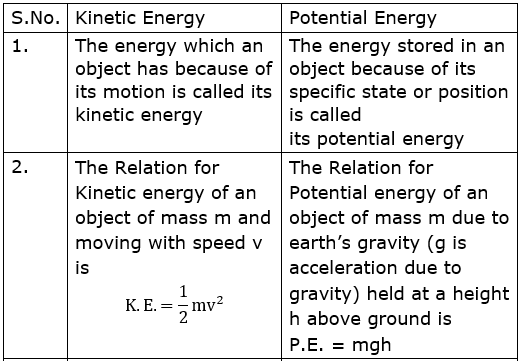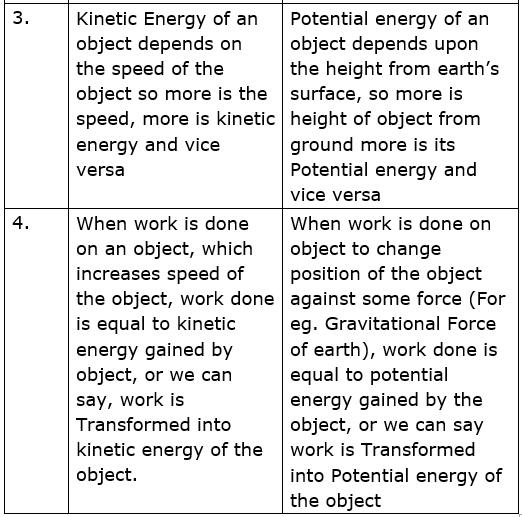Question 2.

Derive the formula for the kinetic energy of an object of mass m, moving with velocity v.

Let us say an object of mass m is moving with speed v, so energy which the object has because of its motion or speed is its kinetic energy.

Now, let us assume object was initially at rest, some work was done on it to increase its speed to v and it gained kinetic energy.

Now from law of conservation of energy, we know kinetic energy will be equal to work done on the object.

Suppose F force was applied on an body of mass m , initially at rest to increase its speed to v in time t, in this time object also covered distance S.

We know work done W on a body of mass m, which moved a distance S due a force F applied on it is given as

W = F × s

Also, Force applied on the object of mass m moving with acceleration a is given as

F = ma

So, work done will be

W = ma× s

We have Newton’s second equation of motion which related displacement S covered by object in time t, having initial velocity u, with its acceleration a, equation is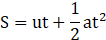Initially object is at rest so, initial velocity is

u = 0 m/s

so, equation becomes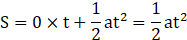So, putting value of displacement s, we get Work done as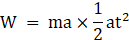This can be further simplified as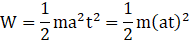Using newton first equation of motion which relates final velocity v gained by object in time t, moving initially with initial velocity u, with acceleration of the object as

v = u + at

Initially object is at rest so, initial velocity is

u = 0 m/s

So, equation becomes

v = 0 + at = at

now, replacing the value of v in above equation to find the work done we get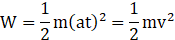Now this work done is equal to kinetic energy of the object of mass m moving with velocity v, i.e. Kinetic energy of the object is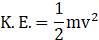Question 3.

Prove that the kinetic energy of a freely falling object on reaching the ground is nothing but the transformation of its initial potential energy.

Let us assume an object of mass m, is kept at rest initially at a height h above the ground, and object is suddenly released , so it will gain speed , and let the Velocity of object just before hitting the ground be v

The situation has been shown in figure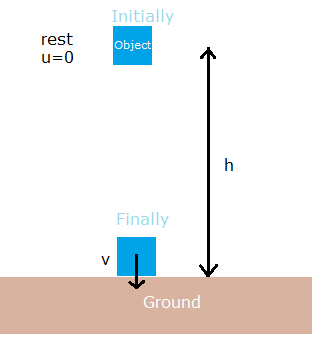Initially object of mass m was at a height h above the ground so potential energy of the object is

P.E. = mgh

g is acceleration due to gravity

now when object approaches ground, just before hitting the ground let the velocity of object be v, so its kinetic energy will beNow , applying newton’s third equation of motion for motion of object

v2 – u2 = 2aS

Here v is final velocity of object,

u is the initial velocity of the object,

S is the displacement covered by object and

a is its acceleration during motion

Now initially object was at rest, so its initial velocity

u = 0 m/s

the object is coming downwards due to gravitational force applied by earth, so it’s acceleration is g, i.e. acceleration due to gravity i.e.

a = g

due to acceleration final velocity of object changed to v, in moving from height h to ground, so its displacement is

S = h

And putting the values in the equation we get

V2 – 02 = 2gh

Or we get value of square of final velocity as

v2 = 2gh

Now, putting the value of v2 in equation of final kinetic energy of the object we get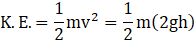Solving we get final kinetic energy of the object as

K.E. = mgh

Initial potential energy of the object is

P.E. = mgh

Now we can see final kinetic energy of the object is same as the initial potential energy of the object

And since initially object was at rest, so its initial kinetic energy was zero, and finally object was just near the ground i.e. its height from surface of earth is zero, so its final potential energy is also zero

This conveys that all the kinetic energy ofa freely falling object on reaching the ground is nothing but the transformation of its initial potential energy

Question 4.

Determine the amount of work done when an object is displaced at an angle of 300 with respect to the direction of the applied force.

Suppose an object is displaced from its original position due to application of a force which is not in the direction of force, force is making an angle of 300 with the direction of displacement

Situation has been shown in figure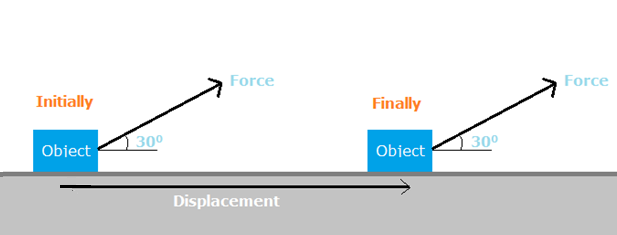Now we know work done on an object due to a force is given by relation

W = FSCos𝜽

Where W is the work done due to force F in displacing object by S , 𝜽 is the angle between force and displacement.

Here let the force applied be F, and displacement be S, angle between force and displacemnt is

𝜽 = 300

So, work done will be

W = FSCos300

From trigonometry we know value of Cos300 as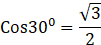So, putting the value we get work done as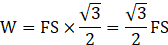So, amount of work done when an object is displaced at an angle of 300 with respect to the direction of the applied force is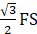Where F is magnitude of applied force and S is the displacement of the object.

Question 5.

If an object has 0 momentum, does it have kinetic energy? Explain your answer.

If an object has 0 momentum then its kinetic energy is also 0, i.e. it does not have kinetic energy

We know momentum of an object is given as

P = mv

Where P is the momentum of object of mass m moving with speed v

Now, P = 0

i.e. mv = 0

So, either mass of object is 0, or its velocity is 0, i.e. it is at rest.

Now object will have some mass let it be m, so its velocity is 0

v = 0

now kinetic energy of any object is given asWhere m is the mass of the object moving with velocity v

Putting v = 0 in the above equation we get kinetic energy as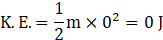So, we get kinetic energy of the object as 0 J , so it does not have kinetic energy if momentum of the object is 0

Question 6.

Why is the work done on an object moving with uniform circular motion zero?

In uniform circular motion work done on an object is zero, because force acting on the object is always perpendicular to its velocity and displacement.

In uniform circular motion,

i. The speed of the object is same throughout but the velocity is changing because the direction of motion of object is continuously changing.

ii. The object is accelerating, and acceleration is towards the center of circular path in which object is moving do force is also acting towards the center of the circular path i.e. perpendicular to the direction of motion.

The Path of circular motion of the object, direction of its velocity, displacement, acceleration and Force at any instant has been shown in the figure :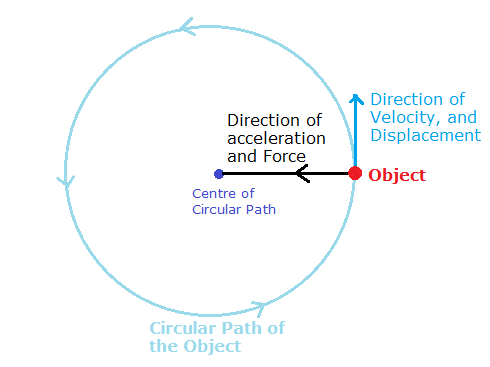As we can see direction of Velocity and displacement at any instant is along the tangent of circle and is perpendicular to direction of acceleration and force i.e. angle between force and displacement at any instant is 900.

Now we know work done on an object due to a force is given by relation:

W = F× s × cos𝜽

Where W is the work done due to force F in displacing object by S , 𝜽 is the angle between force and displacement.

Here let the force applied be F, and very dmall displacement at any instant be S, angle between force and displacemnt is

𝜽 = 900

So, work done will be

W = FSCos900

From trigonometry we know value of Cos900 as

Cos 900 = 0

So, putting the value we get work done as

W = FS × 0 = 0

Work done on an object moving with uniform circular motion is zero

Question 7.

Choose one or more correct alternatives.

For work to be performed, energy must be ….

i transferred from one place to another

ii concentrated

iii transformed from one type to another

iv destroyed

Option (iii) transformed from one type to another

Explanation: For work to be performed, energy must be transformed from one type to another though total energy remain same during this transformation.

Example, if we have to fill an tank overhead at some height with water, we will use electrical energy which will run motor and transfer it into mechanical energy of motor, which will push water towards tank i.e. work is done in displacing water from one place to another and this work is further converted to potential energy of water at some height above the ground , so energy transforms from one type to another in order to perform work.

Question 8.

Choose one or more correct alternatives.

Joule is the unit of …

i. force

ii. work

iii. power

iv. energy

Option(ii) Work , (iv) Energy

Explanation:

We know work done W on a body, which moved a distance S due a force F applied on it is given as

W = FS

SI unit of work done is Joules (J) so 1 J work is said to be done when 1N force is applied on a body and moves it by 1m

i.e. 1 J = 1N × 1m

now work and energy can be interconverted and as we know work done on a body against some external force to change position of body is Potential energy , and work done to increase the speed of the body is called kinetic energy. So energy of a body is equivalent to some type of work done, i.e. work and energy have same unit Joule

Unit of force is Newton (N) and unit of power is watt (W)

Question 9.

Choose one or more correct alternatives.

Which of the forces involved in dragging a heavy object on a smooth, horizontal surface, have the same magnitude?

i. the horizontal applied force

ii. gravitational force

iii. reaction force in vertical direction

iv. force of friction

Option(ii) gravitational force, Option(iii) reaction force in vertical direction

Explanation:

When an object is dragged on any surface it experiences four forces, the Horizontal applied force, frictional force in direction opposite to the applied force, Gravitational force in vertically downward direction due to earth’s gravity and Reaction force due to surface in vertically upward direction.

All the forces have been shown in figure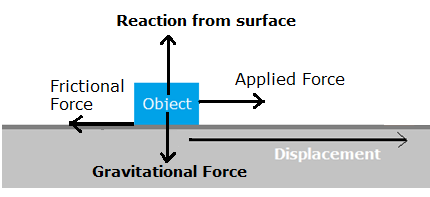Gravitational force and reaction due to Surface are cancelling each other as there is no displacement in vertical direction, i.e. forces are balanced in vertical direction, so their magnitude should be same and direction should be opposite as is the case. Frictional force should be less than applied force for the box to move or start motion.

Question 10.

Choose one or more correct alternatives.

Power is a measure of the ...….

i. the rapidity with which work is done

ii. amount of energy required to perform the work

iii. The slowness with which work is performed

iv. length of time

Option (i) the rapidity with which work is done, Option(iii) The slowness with which work is performed

Explanation:-

Power is define as the rate of doing work or it is the work done per unit time, mathematically power is given as

P = W/t

Here, P is the power when W amount of work is done in time t. so it tells how fast or slow work is being done.

Question 11.

Choose one or more correct alternatives.

While dragging or lifting an object, negative work is done by

i. the applied force

ii. gravitational force

iii. frictional force

iv. reaction force

Option (ii) gravitational force and (iii) Frictional Force

Explanation:

Work done by a force on an object is negative when direction of force acting on object and displacement of object are in exactly opposite direction, or direction of displacement makes 1800 with direction of applied force because we know work done on an object due to a force is given by relation

W = F× s × cos𝜽

Where W is the work done due to force F in displacing object by S , 𝜽 is the angle between force and displacement.

angle between force and displacemnt is

𝜽 = 1800

So, work done will be

W = F× s × cos1800

From trigonometry we know, cos1800 = -1

So putting the value we get work done as

W = -FS

i.e. work done is negative when direction of displacement and applied force are opposite

Now when an object is lifted from ground, gravitational force due to earth in acting in downward direction and displacement is in upward direction due to applied force

as Shown in figure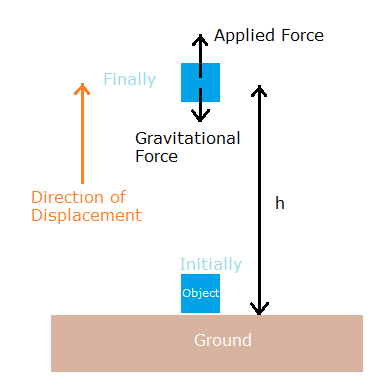Since Gravitational force and direction of displacement are in opposite direction work done by gravitational force is negative

Now when an object is dragged Frictional force is trying to oppose the motion and hence acts in direction of applied force and displacement

As shown in figure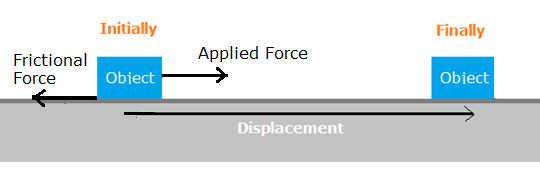As displacement of object and applied frictional force are in opposite direction work done by frictional force is negative.

Question 12.

Rewrite the following sentences using proper alternative.

The potential energy of your body is least when you are …..

i. sitting on a chair

ii. sitting on the ground

iii. sleeping on the ground

iv. standing on the ground

Option(iii) sleeping on the ground

Explanation: Potential energy of any object depends upon its height from the surface of earth, We know the Relation for Potential energy of an object of mass m due to earth’s gravity(g is acceleration due to gravity) held at an height h above ground is

P.E. = mgh

So more is the height of body from ground more is the potential energy and less is the height of body from ground less is the potential energy

Now in case of human body height of body from ground is measured from center of mass of the body which is the point where all mass of the body is assumed to be concentrated , the position of which is near the stomach in human body , so potential energy of body will be least in case of a person sleeping because in that situation height of centre of mass of the body will be minimum from ground.

Question 13.

Rewrite the following sentences using proper alternative.

The total energy of an object falling freely towards the ground …

i. decreases

ii. remains unchanged

iii. increases

iv. increases in the beginning and then decreases

Option (ii) remains unchanged

Explanation: Total energy of a body is sum of kinetic energy and potential energy, kinetic energy depends upon velocity of body, potential energy depends upon position of body(height from surface of earth in gravitational potential energy), they keep varying but their sum i.e. total energy always remains constant, potential energy and kinetic energy keeps on interconverting into each other but the total energy is conserved or unchanged as in accordance with law of conservation of energy, so in case of a freely falling body, speed of body keeps on increasing i.e. kinetic energy is increasing but height of body from ground is decreasing i.e. its potential energy keeps on decreasing , increase in kinetic energy is equal to decrease in potential energy , i.e. the net sum , Total energy of the object remains Same.

Question 14.

Rewrite the following sentences using proper alternative.

If we increase the velocity of a car moving on a flat surface to four times its original speed, its potential energy….

i. will be twice its original energy

ii. will not change

iii. will be 4 times its original energy

iv. will be 16 times its original energy.

Option(ii) will not change

Explanation:

Potential energy of an object does not depend upon its speed but rather its depends on height of object of earth’s surface,its mass and , acceleration due to gravity g , which are constant i.e. does not change during motion of a car on a smooth road as We know the Relation for Potential energy of an object of mass m due to earth’s gravity(g is acceleration due to gravity) held at an height h above ground is

P.E. = mgh

i.e. more is the height of body from ground more is the potential energy and less is the height of body from ground less is the potential energy

now here height of car from ground is constant so potential energy is also same or will not change.

Question 15.

Rewrite the following sentences using proper alternative.

The work done on an object does not depend on ….

i. displacement

ii. applied force

iii. initial velocity of the object

iv. the angle between force and displacement.

Option(iii) initial velocity of the object

Explanation:

we know work done on an object due to a force is given by relation

W = FSCos𝜽

Where W is the work done due to force F in displacing object by S in the direction of force, 𝜽 is the angle between force and displacement

Now we can see here work done is independent of initial velocity of the object , so if a body is moving with any velocity or even if is at rest if , same amount of displacement is caused with same amount of applied force, same amount of work will be done.

Question 16.

Study the following activity and answer the questions.

1. Take two aluminium channels of different lengths.

2. Place the lower ends of the channels on the floor and hold their upper ends at the same height.

3. Now take two balls of the same size and weight and release them from the top end of the channels. They will roll down and cover the same distance.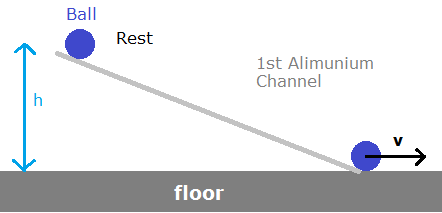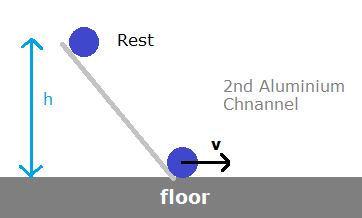Questions

At the moment of releasing the balls, which energy do the balls have?

now suppose two aluminium channels of different height are placed and inclined on a floor such that top of both is that same height, as shown in the figure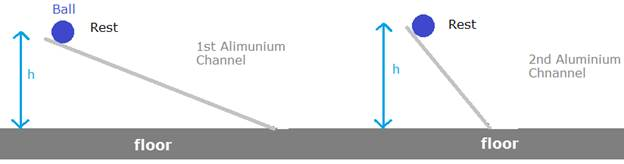Now initially both balls are at rest so they do not have any kinetic energy, and both are at some height above the floor, i.e. earth’s surface, so they will have gravitational potential energy as we know the Relation for Potential energy of an object of mass m due to earth’s gravity (g is acceleration due to gravity) held at an height h above ground is

P.E. = mgh

Question 17.

Study the following activity and answer the questions.

1. Take two aluminium channels of different lengths.

2. Place the lower ends of the channels on the floor and hold their upper ends at the same height.

3. Now take two balls of the same size and weight and release them from the top end of the channels. They will roll down and cover the same distance.As the balls roll down which energy is converted into which other form of energy?

Now as the balls roll down they will gain velocity and height of the balls above the floor will decrease, i.e. potential energy of the ball will be decrease and kinetic energy will increase as they roll down with velocity v as shown in figure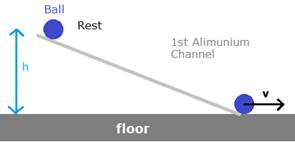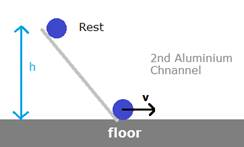Now kinetic energy of the balls of mass m moving with velocity v will be

K.E. = � mv2

Question 18.

Study the following activity and answer the questions.

1. Take two aluminium channels of different lengths.

2. Place the lower ends of the channels on the floor and hold their upper ends at the same height.

3. Now take two balls of the same size and weight and release them from the top end of the channels. They will roll down and cover the same distance.Why do the balls cover the same distance on rolling down?

Now since balls have same initial potential energy as both are at same height above the ground, and all the potential energy is converted into kinetic energy in both the balls, so kinetic energy of both the balls on just reaching the floor will be same , now kinetic energy depends upon velocity of the ball , since kinetic energy of both the balls is same , so both will have same velocity on the floor, with which they are moving forward. Now both ball will stop after covering some distance due to friction, but since their initial velocity on floor is same they will cover equal distances before stopping.

Question 19.

Study the following activity and answer the questions.

1. Take two aluminium channels of different lengths.

2. Place the lower ends of the channels on the floor and hold their upper ends at the same height.

3. Now take two balls of the same size and weight and release them from the top end of the channels. They will roll down and cover the same distance.What is the form of the eventual total energy of the balls?

Total energy of the ball is sum of kinetic energy and potential energy, kinetic energy depends upon velocity of body, potential energy depends upon position of ball (height from surface of earth in gravitational potential energy), they keep varying but their sum i.e. total energy always remains constant.

Now eventually initially ball was at rest at a height h above the surface of earth so form of energy was potential energy now as we know the Relation for Potential energy of an object of mass m due to earth’s gravity (g is acceleration due to gravity) held at an height h above ground is

P.E. = mgh

Question 20.

Study the following activity and answer the questions.

1. Take two aluminium channels of different lengths.

2. Place the lower ends of the channels on the floor and hold their upper ends at the same height.

3. Now take two balls of the same size and weight and release them from the top end of the channels. They will roll down and cover the same distance.Which law related to energy does the above activity demonstrate? Explain.

i. The above experiment law explains law of conservation of energy which states ‘Energy can neither be created nor destroyed. It can be converted from one form into another.

ii. Thus, the total amount of energy in the universe remains constant’.

iii. As we can see here potential energy of both the ball have transferred into kinetic energy and also , kinetic energy of both the balls upon reaching ground was same since their speed was same as they travelled equal distances, this indicates in both cases the initial potential Energy (Same for both as both were at same height) have been converted to kinetic energy and amount of total energy is same for both the balls , and energy has been interconverted from potential to kinetic in both the cases by same amount i.e. it can be generalized that total energy remained constant throughout.

Question 21.

Solve the following examples.

An electric pump has 2 kW power. How much water will the pump lift every minute to a height of 10 m?

Note: Here, electric pump will consume electrical energy , convert it to mechanical energy which will do work on water in raising its height and increasing potential energy of water, i.e. finally all energy is converted to potential energy of water.

Power of the pump is 2 kW i.e. 2000 W of Electrical energy is being consumed every second by motor, we have to find water lifted each minute i.e. energy consumed by motor in 1 minute will be equal to potential energy gained by water in raising to a height of 10 m

We know energy consumed is given as

E = P × t

Where E is the energy consumed by Pump in J, P is the power of Pump in Watt (W), t is the time for which Pump is operated in seconds (s)

Here power of the pump is

P = 2000 W

We want to obtain energy consumed in one 1 minute, so time is

t = 1 min = 60 s

so the energy consumed is

E = 2000 W × 60 s = 120000 J

Now this much energy will be transferred as potential energy of some amount of water

We know the Relation for Potential energy of an object of mass m due to earth’s gravity(g is acceleration due to gravity) held at an height h above ground is

P.E. = mgh

Value of acceleration due to gravity,

g = 9.8 m/s2

Suppose mass of water transferred is m

Height to which water is transferred is

h = 10m

so potential energy of transferred water is

P.E. = m × 9.8 m/s2 × 10m = 98m J

Now equating potential energy of water of mass m equal to energy supplied by pump

P.E. = E

98m = 120000

Solving we get mass of water transferred as

m = 120000/98 Kg = 1224.489 Kg

i.e. around 1224.5 Kg of water is being lifted to height of 10 m by the pump.

Question 22.

Solve the following examples.

If a 1200 W electric iron is used daily for 30 minutes, how much total electricity is consumed in the month of April?

Unit or kilowatt hour kWh is commercial unit of Energy, measured mainly in case of electrical appliances, if an appliance of 1 kW or 1000 W(Spending 1000 J of energy per second) is operated for 1 hour then 1kWh of energy or 1 unit of energy is used

So energy consumed in unit is given as

E = P × t

Where E is the energy consumed by appliance, P is the power of appliance in Kilo Watt (kW), t is the time for which appliance is operated in hours

Here power of the appliance is

P = 1200 W

Converting it to kW

1 kW = 1000 W

i.e. 1 W = 1/1000 kW, so power is

P = 1200 × 1/1000 kW = 1.2 kW

Time for which appliance is used daily is 30 min

1 hour = 60 min

i.e. 1 min = 1/60 hour

i.e. appliance is used daily for

30 × 1/60 hour = � hour

Appliance is used daily in month of April i.e. for 30 Days

So time for which appliance is used is

t = � hour × 30 = 15 hours

putting value of P in t in equation to find energy consumed

E = 1.2 kW × 15 hours = 18 kWh

i.e. 18 kWh or 18 Unit of energy in form of electricity has been used in month of April

Question 23.

Solve the following examples.

If the energy of a ball falling from a height of 10 metres is reduced by 40%, how high will it rebound?

when a ball is kept at some height it gains potential energy, which is its total energy and when it is released just before hitting the ground all its potential energy is converted to kinetic energy, now when it collides with ground if no loss of energy is there , kinetic energy of ball remains same as of before collision but direction of velocity is reversed , now ball goes upward and its magnitude of velocity keeps on decreasing due to gravitational force in downward direction and finally ball stops attaining original height, where again all the kinetic energy is converted to potential energy but if energy is lost during collision , the initial speed with which ball starts moving upward is reduced as kinetic energy is reduced and ball attains less height than before

Ball is initially at a height , h = 10 m above the ground

After collision let the height of ball above the ground be x

Situation has been shown in the figure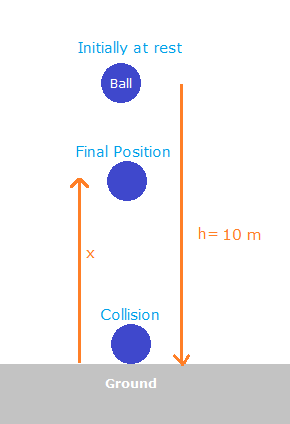Let the mass of ball be m now we know ,The Relation for Potential energy of an object of mass m due to earth’s gravity(g is acceleration due to gravity) held at an height h above ground is

P.E. = mgh

Let initial potential energy of object be Pi, so putting value of h we get initial potential object of ball as

Pi = mg × 10m = 10mg

Now all the potential energy is converted to kinetic energy so kinetic energy of ball just before collision is

Ki = 10mg

After collision kinetic energy or total energy is reduced by 40% , i.e. the kinetic energy just after collision Kf is 60% of the initial energy i.e.

Kf = 60/100 × 10mg = 6mg

Now ball will move up with this kinetic energy upto a height x till all its kinetic energy is converted to potential energy and comes to rest momentarily before again falling downwards

Now final potential energy of the ball at a height x above the groung will be

Pf = mgx

Now, equating final potential energy equal to the kinetic energy of the ball just after collision i.e.

Pf = Kf

i.e. mgx = 6mg

solving we get

x = 6m

So the ball rebounds upto a height of 6m above the ground

Question 24.

Solve the following examples.

The velocity of a car increase from 54 km/hr to 72 km/hr. How much is the work done if the mass of the car is 1500 kg ?

Now here velocity of a car is increased i.e. car is accelerated which means some force is applied on the car and since car is in motion and accelerating so it also travelled some displacement from original position

Situation has been depicted in the figure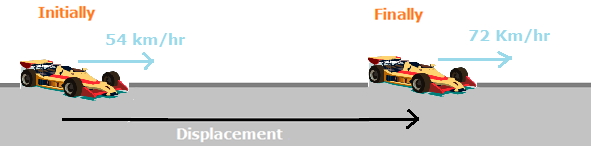Now we know force on a body is given as

F = ma

Where F force is applied on a body of mass m, to move it with acceleration a

we know work done on an object due to a force is given by relation

W = FS

Where W is the work done due to force F in displacing object by S in the direction of force

So putting the value of force in above equation we get the magnitude of work done as

W = FS = maS

Mass of car is given

m = 1500 kg

Now, finding the value of (aS)

We know newton’s third equation of motion which is

v2 – u2 = 2aS

Here v is final velocity of object, u is the initial velocity of the object, S is the displacement covered by object and a is its acceleration during motion

here velocities are given in Km/hr , converting them to SI i.e. m/s

we know , 1 Km = 1000 m

1 hour=60 minute,1 minute = 60 sec

So, 1 hour = 60 × 60 = 3600 sec

So we get,

1 km/hr = 1000 m/3600 s

=10/36 = 5/18 m/s

Now here initial velocity of the car is

u = 54 km/hr = 54 × 5/18 = 15 m/s

final velocity of the Car is

v = 72 km/hr = 72 × 5/18 = 20 m/s

putting values of v and u in above equation we get

(20ms-1)2 - (15ms-1)2 = 2×aS

i.e.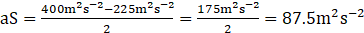so putting the value of mass of car and value of aS in equation of work done we get work done as

W = maS = 1500kg × 87.5 m2s-2 = 131250 J

So the amount of work done is 131250 J

Question 25.

Solve the following examples.

Ravi applied a force of 10 N and moved a book 30 cm in the direction of the force. How much was the work done by Ravi?

Here a book is displaced by 30 cm due to 10 N force, as depicted in the figure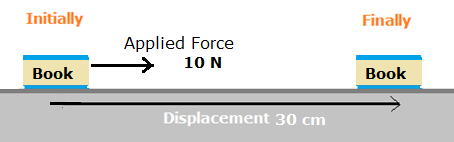we know work done on an object due to a force is given by relation

W = FS

Where W is the work done due to force F in displacing object by S in the direction of force.

Here the force applied is

F = 10 N

The displacement of book in the direction of force

S = 30 cm

All units should be in SI so converting cm to m

1 cm = 1/100 m

So we get displacement as

S = 30 × 1/100 = 30/100 m = 0.3 m

So work done on the book is

W = 10 N × 0.3 m = 3 N

i.e. Ravi did 3 J work to move the book

Question 26.

Study the various instances of transformation of energy you see around you and discuss them in class.